# Systematics of beta decayThe Feynman diagram for the beta decay of a neutron into a proton, electron, and electron antineutrino via an intermediate heavy W- boson
Beta decay is quite a bit more subtle and complex than the other two common forms of radioactivity—alpha decay and gamma decay. In alpha decay, nucleons fall out of a nucleus because they are electrically repelled by the other protons and can go into a lower energy state by leaving, while in gamma decay nucleons fall down into a lower energy state (under the rules of quantum mechanics) in a similar manner to the drop in electron energy levels in an atom, albeit at a much more energetic level.

Beta decay, unlike the other two, involves actual transmutation of nucleons, typically among protons, neutrons, and electrons/positrons. This has far-reaching consequences involving electric charge and various conservation laws. This article will attempt to peel back the layers of complexity one at a time.

Beta decay involves the "weak force", unlike alpha decay, which involves the "strong force". Nevertheless, beta decay has some extremely far-reaching consequences for radioactivity, nucleosynthesis, and supernovas.

The weak force is one of the four fundamental forces currently believed to exist:

• the gravitational force
• the electromagnetic force
• the weak force
• the strong force

The electric and magnetic forces were once considered to be separate, but they were unified so straightforwardly in the late 19th century that they are no longer treated as separate. The electromagnetic and weak forces were unified in the late 1960s, into the "electroweak force", but that connection is sufficiently esoteric that it has not supplanted the separate electromagnetic and weak forces. There are "grand unified theories" unifying the last three of these forces, and the physics community continues to search for a "theory of everything" that brings gravity into the picture. For all practical purposes, the four forces listed above are generally considered the fundamental forces.

The first two of these forces are well known without specialized knowledge of nuclear physics, mostly because they operate at macroscopic distances. The last two only operate at microscopic distances (about 10−15 meters, or the diameter of a proton), so they are limited to subatomic particles.

• Q: Why are they called "forces"? What is doing the pushing?
• A: The term "force", applied to the gravitational and electromagnetic forces, is obviously reasonable. Also, the strong force could sort of be thought of as a force, making nucleons stick together. It takes a certain amount of mechanical pressure to pull nucleons out of a nucleus. But the weak "force" is hard to visualize as a force. Nevertheless, all four of these interactions are called "forces" for historical consistency.

## The common transformations

The weak force acts on just abut every particle that exists—baryons (protons and neutrons), hadrons (various other things made of quarks), leptons (electrons, neutrinos, etc.), bosons, and so on. For example, the interaction shown in the above right corner shows weak interactions involving up and down quarks, a "weak vector boson", an electron, and an antineutrino. For all but extremely esoteric analysis of fundamental particle physics, hardly any of that matters. What's important is that a neutron turned into ("decayed into") a proton, and electron and an antineutrino. The various quarks, and the vector boson underwent beta transformations, but, in a simpler sense, we can just say that the neutron underwent a beta transformation. The interaction shown in the figure is the first of these: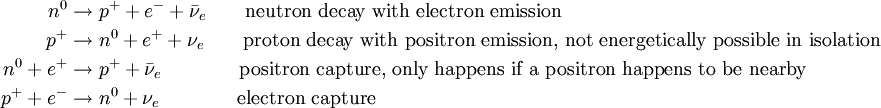The first of these is classical "beta decay", that is, the emission of a "beta ray", first discovered in the earliest days of radioactivity research. The "beta rays" were soon identified as being just electrons.

The second is generally called "beta plus" decay, the positron being considered a "beta plus ray". That, and the fourth one, electron capture, are beta transformations that occur for many isotopes.

It is common to use the Greek letter "β" instead of "e" for the electron, still using the plus or minus superscript. Also, the charge symbol for protons and neutrons is often omitted. So the four equations above become: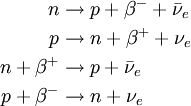The "e" subscript on the neutrino is still used, to distinguish electron-type neutrinos from muon-type and tau-type.

## The reverse transformations

All physical processes are supposedly reversible in time, so that, for example, if an alpha particle is thrown at a 222Rn86 nucleus with just the right energy, it could undergo "reverse alpha decay", producing a 226Ra88 nucleus. Or an antineutrino could combine with and electron and a proton with just the right energy to produce a neutron: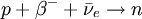But this is a statistical impossibility—it would require that the particles be in exactly the right place and with exactly the right energy. It is in the same category as heat flowing uphill—a violation of the Second Law of Thermodynamics.

## Antiparticles

These transformations work on antiparticles also. Note that the electron ("beta-minus particle") and positron ("beta-plus particle") are antiparticles. So, in principle, we could have: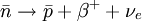## Conservation laws

When dealing with beta transformations, one needs to take note of various conservation laws. (For alpha and gamma transformations, particles don't change, so these issues don't come up.)

• Charge - this is the electric charge. So a neutron, with zero charge, can decay into a proton (+1), and electron (-1), and a neutrino (zero).
• Baryon number - Baryons are "big" nucleons. For our purposes, they are protons and neutrons. They can't just go away. Nor can they transform into their antiparticles. (A proton and antiproton annihilating each other would satisfy this, since the baryon numbers are +1 and -1, but that transformation is beyond the scope of this article.)
• Lepton number. The lepton numbers can be thought of as "electron-ness", "mu-ness", and "tau-ness". Electrons and positrons have electron-ness of +1 and -1 respectively (and zero for the other two). Here is where the neutrinos come into play. An electron neutrino has an electron-ness of +1, while and anti-neutrino has -1. That's why the neutron-to-proton-and-electron transformation needs to create the antineutrino to carry off the -1, compensating for the +1 of the electron.

## The general principle

With these preliminaries out of the way, the general principle can be stated:

Any beta transformation will happen, with some probability, if the conservation laws are satisfied and the masses of the results add up to less than the masses of the original particle(s). If it can happen, it will happen.

The mass of the neutron is 1.008664916 amu, that of the proton is 1.007276467 amu, and that of the electron is .000548579909070 amu. (The pass of the antineutrino is negligible.) The lost mass is .00083986909093, which is carried off in the kinetic energy of the result particles, about 0.78 MeV, in accordance with E=mc².

• Q: The neutron is unstable and decays? Why don't all atoms fall apart?
• A: Yes, a neutron, standing alone, is unstable. It has a half-life of about 15 minutes. To see why this doesn't happen for neutrons inside a nucleus, keep reading.

The reverse transformation, turning a proton into a neutron, positron, and neutrino, has a negative energy budget, so it does not occur. That is, it does not occur in isolation. But when the proton is in a nucleus, the energy situation of the entire nucleus must be considered, and this transformation can occur for certain isotopes. It is "beta plus" decay. It is a very rare type of decay of natural Potassium-40, and can occur in some radioactive isotopes. The most notable of these is artificially-prepared Oxygen-15, used in "PET" (positron emission tomography) scans.

## Given that something can happen, how often does it happen?

The probability, per unit time, of a beta transformation happening, varies enormously, and determines the substance's half-life. This probability is determined by how "close" the particles get to each other. They must be extremely close—the weak force is weak, and only acts over a distance of about the size of a nucleon, or 10−15 meter. This distance is measured in terms of the size of the quantum mechanical wave function. It can actually be quite large (compared with the size of a nucleon) in electron capture, because electrons typically have a rather dispersed wave function.

• Q: How about neutron decay? How can the closeness of approach be theorized for a single particle?
• A: In such cases it is necessary to consider the more esoteric theory involving quarks, that this article is ignoring for the most part. In the diagram above, two quarks collided within the neutron, initiating the decay. One can think of larger particles like neutrons as having quarks rattling around inside, ready to collide.

## Half-life

The probability of a given transformation, within a given configuration of particles, per unit time, is fixed. This means that the decay proceeds exponentially. (This is true of alpha and gamma decay also, for the same reason.) This gives every isotope a characteristic half-life. Half-lives are usually not able to be calculated on a theoretical basis; they are measured from observation. The probabilities derive from the overlap of the wave functions, leading to quantum mechanical probability. This is fixed, by the rules of quantum mechanics.

## Why neutrons are usually stable when inside a nucleus

Neutrons, by themselves, decay. This follows from the mass difference between the neutron and the resultant proton and electron. But, when the entire nucleus is taken into account, it's often a different story.

Consider the decay of Argon-39 to Potassium-39. This is a standard beta-minus decay. This Argon atom has 18 protons and 21 neutrons, and an nuclear mass of 38.95443856 amu. The Potassium atom has 19 protons and 20 neutrons, and a nuclear mass of 38.95328347 amu. The beta-minus particle (electron) ejected by the nucleus weighs .00054858 amu. So the total mass decrease is .000606513 amu, so the decay is energetically possible, releasing .000606513 amu of equivalent energy.

Now consider the hypothetical beta-plus decay of Argon-37 to Chlorine-37. This Argon atom has 18 protons and 19 neutrons, and a nuclear mass of 36.9569019 amu. The Chlorine atom has 17 protons and 20 neutrons, and a nuclear mass of 36.9565767 amu. The beta-plus particle (positron) ejected by the nucleus weighs .00054858 amu. There would be an overall mass increase of .00022343 amu. This decay cannot happen.

But suppose that, instead of releasing a positron, the excess electron from the atom's electron cloud simply went down into the nucleus. The nuclear transformation would be: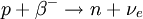This would release .0008737 amu of mass. It is an electron capture event. This decay occurs with a half-life of 35 days.

• Q: If neutrons weigh more than protons, why does a beta-minus event that turns a neutron into a proton sometimes increase the mass, and hence the energy?
• A: There is a contribution to the nuclear mass from the electric repulsion force of the protons. Adding a proton increases that repulsion. This, by the way, is why elements other than the very lightest have more neutrons than protons.

## The curious case of Beryllium-7

Radioactive decay rates are very stable and repeatable. In fact, this phenomenon was remarked upon by Henri Becquerel in 1896, almost immediately after discovering radioactivity. Prior to that time, all properties of elements (other than their atomic weight, which has to be constant due to conservation of mass) were dependent on the chemical state. An element's color, solid/liquid/gas state, oxidation/reduction state, and so on, all depended on what chemical compound it was in. But Becquerel noticed that the radioactivity of Uranium remained constant no matter what its chemical state.

The reason is that all those properties are properties of the electron cloud. Radioactivity comes from the nucleus, far from the electrons. Though of course Becquerel didn't know that. Radioactivity proceeds from the properties of the quantum mechanical wave function, which depends on the Schrödinger equation. The electron cloud has almost no effect on this.

Electron capture events are the exception to this. The probability of an electron capture depends on the availability of electrons in the vicinity of the nucleus. Things that change the location of electrons can change the radioactive decay rate.

In fact, if the atom is completely ionized, the electron-capture decay rate essentially goes to zero and the half-life essentially goes to infinity. Furthermore, the normal beta-minus decay rate can change because it becomes easier for ejected electrons to leave the vicinity of the nucleus. Ionizing Rhenium-187 has been observed to accelerate its decay by a factor of one billion. This effect was produced through the use of a particle accelerator. It does not occur in nature.

Electron capture events generally involve the innermost electrons, in the "1s" orbital. Chemical properties (oxidation, reduction, etc.) involve the "valence" electrons in the outermost shell. So chemical effects on radioactivity are almost never observed.

But there is a well-known exception to this. Beryllium, with an atomic number of 4, has only two electrons in the "1s" orbital and two in the "2s" valence orbital. The latter electrons are so close to the nucleus that chemical changes can have a small but measurable effect on the decay rate. Beryllium-7 is an electron-capture nucleus, decaying into Lithium-7 with a half-life of 53 days. This half-life can vary by up to 1.5% due to the chemical state. Creationist websites often appear to attach great significance to this situation and the one described above about Rhenium-187, even though they do not arise in radiometric dating, perhaps in an attempt to downplay the reliability of radiometric dating.